#### Backprojections

Erdmann proposed a practical way to compute effective motion commands by separating the reachability and recognizability issues [311,312]. Reachability refers to characterizing the set of points that are guaranteed to be reachable. Recognizability refers to knowing that the subgoal has been reached based on the history I-state. Another way to interpret the separation is that the effects of nature on the configuration transitions is separated from the effects of nature on sensing.

For reachability analysis, the sensing uncertainty is neglected. The notions of forward projections and backprojections from Section 10.1.2 can then be used. The only difference here is that they are applied to continuous spaces and motion commands (instead of). Let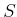denote a subset of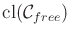. Both weak backprojections,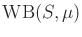, and strong backprojections,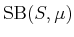, can be defined. Furthermore, nondirectional backprojections ,and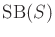, can be defined, which are analogous to (10.25) and (10.26), respectively.

Figure 12.46 shows a simple problem in which the task is to reach a goal edge with a motion command that points downward. This is inspired by the peg-in-hole problem. Figure 12.47 illustrates several backprojections from the goal region for the problem in Figure 12.46. The action is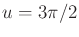; however, the actual motion lies within the shown cone due to nature. First suppose that contact with the obstacle is not allowed, except at the goal region. The strong backprojection is given in Figure 12.47a. Starting from any point in the triangular region, the goal is guaranteed to be reached in spite of nature. The weak backprojection is the unbounded region shown in Figure 12.47b. This indicates configurations from which it is possible to reach the goal. The weak backprojection will not be considered further because it is important here to guarantee that the goal is reached. This is accomplished by the strong backprojection. From here onward, it will be assumed that backprojection by default means a strong backprojection. Using weak backprojections, it is possible to develop an alternative framework of error detection and recovery (EDR), which was introduced by Donald in .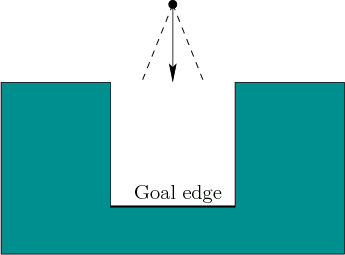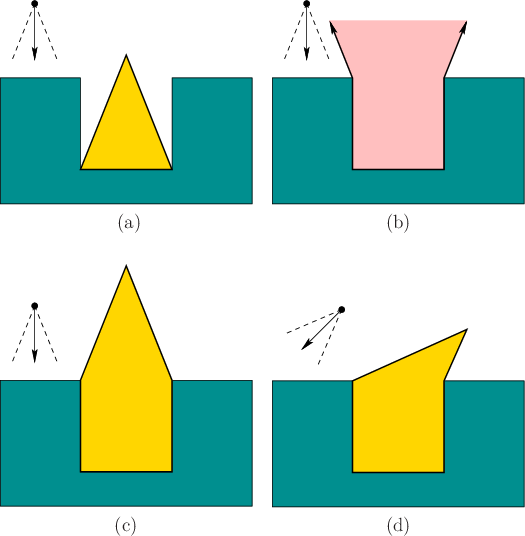Now assume that compliant motions are possible along the obstacle boundary. This has the effect of enlarging the backprojections. Suppose for simplicity that there is no friction (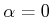in Figure 12.44a). The backprojection is shown in Figure 12.47c. As the robot comes into contact with the side walls, it slides down until the goal is reached. It is not important to keep track of the exact configuration while this occurs. This illustrates the power of compliant motions in reducing uncertainty. This point will be pursued further in Section 12.5.2. Figure 12.47d shows the backprojection for a different motion command.

Now consider computing backprojections in a more general setting. The backprojection can be defined from any subset ofand may allow a friction cone with parameter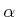. To be included in a backprojection, points from which sticking is possible must be avoided. Note that sticking is possible even if. For example, in Figure 12.46, nature may allow the motion to be exactly perpendicular to the obstacle boundary. In this case, sticking occurs on horizontal edges because there is no tangential motion. In general, it must be determined whether sticking is possible at each edge and vertex of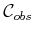. Possible sticking from an edge depends on,, and the maximum directional error contributed by nature. The robot can become stuck at a vertex if it is possible to become stuck at either incident edge.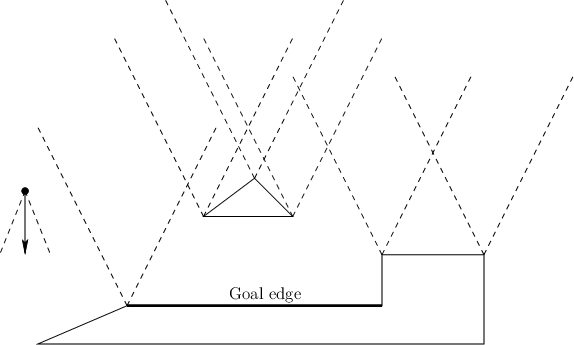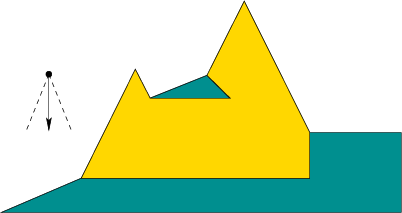Steven M LaValle 2020-08-14